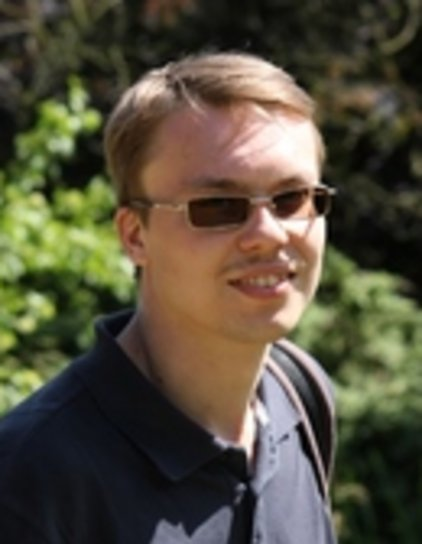# Dr. Sergey Dolgov

Computational Methods in Systems and Control Theory
Max Planck Institute for Dynamics of Complex Technical Systems

### Main Focus

Numerical analysis, linear and multilinear algebra, matrix and tensor analysis, fast algorithms, tensor product formats, dynamical problems, stochastic models

## Education and qualifications

• 2014 PhD in Mathematics. University of Leipzig. Thesis: Tensor product methods in numerical simulation of high-dimensional dynamical problems. magna cum laude.
• 2011 M.Sc. in Applied Mathematics and Physics. Moscow Institute of Physics and Technology. Thesis:  Iterative methods with the use of tensor decompositions. Excellent.
• 2009 B.Sc. in Applied Mathematics and Physics.  Moscow Institute of Physics and Technology. Thesis: Algebraic methods of sparse grids.  Excellent.

## Experience

• 2014-now Postdoctoral Researcher. Max Planck Institute for Dynamics of Complex Technical Systems, Magdeburg, Germany.
• 2011-2014 PhD student. Max Planck Institute for Mathematics in the Sciences, Leipzig, Germany.
• 2008-2011 Junior Researcher. Institute of Numerical Mathematics, Russian Academy of Sciences, Moscow, Russia.

## Software projects

• I am a co-developer of TT-Toolbox: http://github.com/oseledets/TT-Toolbox
An open-source object-oriented MATLAB/C/Fortran package for computations in the Tensor Train and QTT-Tucker tensor product formats. Main features include:
1. Overloaded linear algebra with matrices and vectors in a tensor format.
2. Compression of given data into a format by direct (SVD) and sparse (cross) procedures.
3. A collection of examples with analytical tensor formats (finite difference Laplacian, etc.)
4. Alternating iterative algorithms for solution of linear systems and eigenvalue problems.
• tAMEn: http://github.com/dolgov/tamen
Purely Matlab routines for alternating iterative solution of the ordinary differential equations in the tensor train format.  See the paper "Alternating minimal energy approach to ODEs and conservation laws in tensor product formats", [arXiv:1403.8085].
The main feature of the new algorithm is the spectral accuracy in time and the possibility to conserve linear invariants and the second norm up to the machine (not tensor approximation) precision.
Another difference with TT-Toolbox is the storage scheme, which allows sparse tensor product factors.

## Publications

Peer-reviewed articles
1. Fast tensor product solvers for optimization problems with fractional differential equations as constraintsApplied Mathematics and Computation, 273: 604623, 2015 (with John W. Pearson, Dmitry V. Savostyanov, Martin Stoll).
2. Polynomial Chaos Expansion of Random Coefficients and the Solution of Stochastic Partial Differential Equations in the Tensor Train FormatSIAM/ASA J. Uncertainty Quantification, 3(1): 11091135, 2015 (with B. N. Khoromskij, A. Litvinenko and H. G. Matthies).
3. On evolution of solution times for the chemical master equation of the enzymatic futile cycleRuss. J. Numer. Anal. Math. Modelling30(1): 3742, 2015 (with E. E. Tyrtyshnikov).
4. Simultaneous state-time approximation of the chemical master equation using tensor product formats. Numer. Linear Algebra Appl.22(2):197219, 2015. (With B. Khoromskij)
5.  Computation of extreme eigenvalues in higher dimensions using block tensor train format. Computer Phys. Comm., 185(4):1207–1216, 2014. (With B. N. Khoromskij, I. V. Oseledets and D. V. Savostyanov)
6.  Alternating minimal energy methods for linear systems in higher dimensions. SIAM J Sci. Comput., 36(5):A2248–A2271, 2014. (With D. V. Savostyanov)
7.  Corrected one-site density matrix renormalization group and alternating minimal energy algorithm. In Proc. ENUMATH 2013, 103:335343, 2015. (With D. V. Savostyanov)
8.  Low-rank approximation in the numerical modeling of the Farley-Buneman instability in ionospheric plasma. J. Comp. Phys., 263:268–282, 2014. (With A. P. Smirnov and E. E. Tyrtyshnikov)
9.  Exact NMR simulation of protein-size spin systems using tensor train formalism. Phys. Rev. B, 90:085139, 2014. (With D. V. Savostyanov, J. M. Werner and I. Kuprov)
10.  Two-level QTT-Tucker format for optimized tensor calculus. SIAM J. on Matrix An. Appl., 34(2):593–623, 2013. (With B. Khoromskij)
11.  TT-GMRES: solution to a linear system in the structured tensor format. Russ. J. Numer. Anal. Math. Modelling, 28(2):149–172, 2013.
12.  Superfast Fourier transform using QTT approximation. J. Fourier Anal. Appl., 18(5):915–953, 2012. (With B. N. Khoromskij and D. V. Savostyanov)
13.  Fast solution of multi-dimensional parabolic problems in the tensor train/quantized tensor train–format with initial application to the Fokker-Planck equation. SIAM J. Sci. Comput., 34(6):A3016–A3038, 2012. (With B. N. Khoromskij and I. V. Oseledets)
14.  Low-rank tensor structure of solutions to elliptic problems with jumping coefficients. J. Comput. Math., 30(1):14–23, 2012. (With B. N. Khoromskij, I. V. Oseledets and E. E. Tyrtyshnikov)
15.  Solution of linear systems and matrix inversion in the TT-format. SIAM J. Sci. Comput., 34(5):A2718–A2739, 2012. (With I. V. Oseledets)
16.  A reciprocal preconditioner for structured matrices arising from elliptic problems with jumping coefficients. Linear Algebra Appl., 436(9):2980–3007, 2012. (With B. N. Khoromskij, I. V. Oseledets and E. E. Tyrtyshnikov)
Preprints
1. Low-rank solutions to an optimization problem constrained by the Navier-Stokes equations. MPI MD preprint 15-15, 2015. (with M. Stoll)
2. Low-rank solvers for unsteady Stokes-Brinkman optimal control problem with random data. MPI MD preprint 15-10, 2015. (with P. Benner, A. Onwunta and M. Stoll)
3. Alternating minimal energy approach to ODEs and conservation laws in tensor product formats. arXiv preprint 1403.8085, 2014.

## Conference talks (and 1 poster) in 2015

1. Low-rank cross algorithms for approximations in parametric equations. SIAM conference on Applied Linear Algebra, Atlanta, US, October 28, 2015.
2. Alternating iteration for low-rank solution of linear systems with large indefinite matrices. 5th Workshop Matrix Equations and Tensor Techniques, University of Bologna, Italy, September 21, 2015.
3. Low-rank solution of optimization problems constrained by fractional differential equations. Conference European Numerical Mathematics and Advanced Applications, ODTU, Ankara, Turkey, September 14, 2015.
4. On parallelization of alternating linear schemes for low-rank high-dimensional optimization. Conference Matrix Methods in Mathematics and Applications, Skoltech, Moscow, Russia, August 24, 2015.
5. Low-rank solution of optimization problems constrained by fractional differential equations. Young Investigators Conference, RWTH, Aachen, Germany, July 22, 2015.
6. (Poster) Alternating iteration for low-rank solution of high-dimensional equations. Workshop Low-rank Optimization and Applications, HIM, Bonn, Germany, June 9, 2015.

## Computer skills and languages

• Programming: C/C++, Fortran, MATLAB, MPI, OpenMP, Lapack/MKL.
• Text editing and publishing: Latex, OpenOffice.
• OS: Linux, Windows.
• Russian: Native
• English: C1 (IELTS: 7.5)
• German: Basic
Go to Editor View